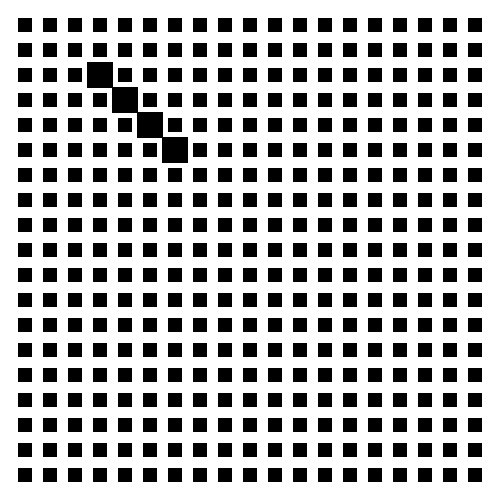# Groups

processing, grid, Law of Similarity, repeat, chance

The law of similarity on action. Elements that are similar in size or shape are seen as a group. Uses nested loops``````fill(0);
//noStroke();
rectMode(CENTER);
size(500, 500);
background(255);
int [] values = {5, 10, 20, 25, 50, 100};
float step = values[floor(random(0, values.length))];
for (float x = step; x < width; x+=step) {
for (float y = step; y < height; y+=step) {
if (x - step == y && x > step*3 && y < step*7) {
rect(x, y, step, step);
} else {
rect(x, y, step/2, step/2);
}
}
}
saveFrame("out.png");
``````